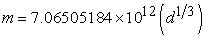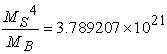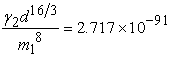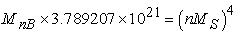﻿ New Discovery about Prediction of a Particle ‘K-Suryon’ as Basic Building Block of Mass

### New Discovery about Prediction of a Particle ‘K-Suryon’ as Basic Building Block of MassOPEN ACCESSPEER-REVIEWED

## New Discovery about Prediction of a Particle ‘K-Suryon’ as Basic Building Block of Mass

Siva Prasad Kodukula1,2,1Adviser Centre for Innovation & Discovery, Bheemunipatnam, India
2Employee of Jindal Steel & Power Ltd, Angul, India

### Abstract

With the help of ‘Heart of the God model of the universe’ ,‘Siva’s classical Equation for space time’  and ‘Siva’s Theory of quantum Gravity’  a new particle ‘K-Suryon’ has been predicted. It is the elementary particle with mass 1.15 x 10-64 kg and radius 8.15 x 10-134 mts. It is described as the basic building block of ‘space- time’ & mass. Mass of real particle ‘K-Suryon’ is 4.6x10-64 Kgs. It has to be incorporated in standard model. This discovery will explain how the Quantum Gravity is synchronizing with General Relativity. It will open new ways of research in ‘dark matter’ and ‘black hole’ physics. It reveals the concept of mass creation more profoundly than ‘Higgs mechanism’.

• Kodukula, Siva Prasad. "New Discovery about Prediction of a Particle ‘K-Suryon’ as Basic Building Block of Mass." International Journal of Physics 2.1 (2014): 12-14.
• Kodukula, S. P. (2014). New Discovery about Prediction of a Particle ‘K-Suryon’ as Basic Building Block of Mass. International Journal of Physics, 2(1), 12-14.
• Kodukula, Siva Prasad. "New Discovery about Prediction of a Particle ‘K-Suryon’ as Basic Building Block of Mass." International Journal of Physics 2, no. 1 (2014): 12-14.

 Import into BibTeX Import into EndNote Import into RefMan Import into RefWorks

### 1. Introduction

As per ‘Heart of God model of the universe’, the size of the universe is constant. Thus there is a limitation to the elastic nature of any electromagnetic wave after which the wave will form in to particle. This basic point concluded to the prediction of most elementary particles which is a basic building block of mass. The Mass creation is interlinked with space, time and space time fluid of the universe and a new theory of Quantum Gravity has been introduced to explain the creation of mass. As per this new concept the space is a singularity and it converts in to space time fluid as per ‘space time –equivalence equation” .This space time fluid converts in to mass and explained the creation with the help of Siva’s Theory of Quantum Gravity’. Here a detailed analysis has been done to describe ‘K-Suryon’ completely.

### 2. Discussion & Calculations

2.1. Mass of K-Suryon

As per Heart of God model , the size of the universe is constant i.e. 1.9147644×1022mts. This will be the maximum wave length of any electromagnetic wave. We know maximum velocity is light. So the least frequency (ν) can be calculated by dividing light velocity with maximum wave length and is equal to 1.5656887×10-14 /sec. So by equations E = mc2 and E = hν We can find its mass as 1.15 ×10-64kgs. Thus there is a limitation to the elastic nature of any electromagnetic wave after which the wave will form in to particle. This basic point concluded to the prediction of most elementary particles which is a basic building block of mass. Mass creation is interlinked with space, time and ‘space- time fluid’ of the universe and a new theory of Quantum Gravity have been introduced to explain the creation of mass. As per this new concept the space is a singularity and it converts in to ‘space time fluid’ as per ‘space time –equivalence equation’ . This space time fluid converts in to mass and explained the creation with the help of ‘Siva’s Theory of Quantum Gravity’. Here a detailed analysis has been done to describe ‘K-Suryon’ completely.

2.2. Mass creation process & Radius of K-Suryon

We have space time equation for mass as-And γd8/3 =1.686656885× 1012

Where ‘γ’ is ‘space time fluid’ density of mass ‘m’ 

•  Therefore density of ‘space -time fluid’ for K-Suryon calculated by the above mentioned space time fluid density equation is 3.3238545×10626 kg/cum

•  K-Suryon is the building block of mass creation .

•  K-Suryon is outcome of singularity point in space time continuum of General relativity.

•  In the beginning, K-Suryon is a point where only space will be available .

•  Thus it will have both the masses defined for singularity MS and the concerned mass of black hole MB and is related by equation(1)

•  K-Suryon is the mass equivalent to the energy of the wave whose wave length is maximum and limited to the size of the universe or Dia of Heart of God. And is calculated as 1.15×10-64kgs

•  By definition of K-Suryon, it is singularity as a center of black hole. So the mass of this singularity (MS) can be calculated by considering the mass 1.15x10-64kgs as its mass of black hole (MB). Thus MS can be calculated as 8.125×10-12 Kgs by equation (1).

•  As per Siva’s Quantum theory of gravity , any mass ‘m1’ will have a space time density levels (Quantized) and the density level at distance‘d’ from its center is ‘γ2’. The relation shown by equation-(2)

•  If we apply the equation to K-Suryon, The ‘d’ will be the radius of K-Suryon. In this equation the mass ‘m’ is the mass of K-Suryon as a mass of black hole with a mass of singularity(MS) at its center.

•  If we substitute γ = 3.3238545x10626 Kg/cum and m = 8.125×10-12 Kgs in equation (2) we can calculate the radius of a single K-Suryon as a singularity i.e d = 8.15×10-134 mts.

This is the starting point of mass creation where space only exists. When one more K-Suryon added to it the space will form space time. But adding K-Suryon is an event which will not occur within 7.6813×10-44sec (time taken to film change of the universe ). Thus the process of adding ‘K-Suryons’ considered as one event which is outcome of complete formation of real particle ‘K-Suryon’.

When two K-Suryons added, the space of K-Suryon will be changed in to ‘space –time’  to start the process of mass creation. But still it is a singularity only. Because this space time fluid will bear a mass but density of that mass is very less than the density of ‘space time fluid’ associated to that mass. This process of adding K-Suryons should be continued so that the created mass will have more density than its ‘space time fluid’. Still it can not be treated as mass .It is a black hole with a singularity at its center only. The process will be continued until the mass acquires a density which is more than its space time fluid density by adding more no of K-Suryons.

Thus the mass will be created by adding K-Suryons. This singularity will exist until the mass will be created. Singularity exist means, it is a black hole. So by equation (1) we can say that black hole will have a mass of singularity ‘MS’ and mass of black hole ‘MB’. Now it is obvious that if two singularities added, mass of singularity will be increased and mass of black hole also will be increased and the space time fluid will start to be created.

Let us suppose ‘n’ K-Suryons added to form a particle of mass.

As calculated above for a single K-Suryon- mass of black hole = MB = 1.15×10-64kgs and mass of its singularity = MS = 8.125 ×10-12 Kgs as per equation (1).

So the mass of singularity for mass created by ‘n’ no.of K-suryons is = n MS

If we substitute ‘n MS’ in place of ‘MS’ in equation (1)

We can find its black hole massStill it is a black hole with diameter d =8.15 x10-134 mts as calculated by equation(2).Thus the mass of black hole MnB =MB =1.15×10-64kgs.

Physically, it means, when a K-Suryon added to another K-Suryon, the mass of singularity of this total entity will be increased and its ‘black hole mass’ also will be increased as per equation no(1). The increment in mass of singularity is not visible only its effect will be visible as increment in mass of black hole. At the same time the mass of singularity cannot be neglected. But what happens to this mass of singularity? This increment in mass of singularity will be resulted as decrement in volume of mass so that the ‘black-hole mass’ will have a density more than its ‘space time fluid’. Thus the density of the ‘black hole mass’ will acquire space time fluid by adding more no of k-Suryons and finally it will emerge as a real particle without a singularity(since the space time fluid will be outside of its mass).Now, it is not a black hole.

The no. of K-Suryons to become a ‘real particle K-Suryon’ that can be observed in this universe can be calculated as-

A finally created real particle K-Suryon will have MB = 1.15x10-64kgs & MS = 8.125×10-12 Kgs.

Therefore 1.15x10-64×3.789207×1021=(n×8.125×10-12)4 implies n=(99.989)1/4=3.162=next whole number=4.

Thus four (4) K-Suryons forms a real particle that exists as mass in this nature. So the least mass that can be observed as a real particle is n×mass of K-Suryon (virtual)=4×1.15×10-64 =4.6×10-64Kgs.

2.3. Why this Concept is More Profound than Higgs Mechanism?

•  Higgs mechanism is based on standard model which describes that the elementary particles are made up of simple unit charge waves  where as K-Suryon concept describes the space time fluid conversion in to mass. Further it can explain that a charge can not be defined for zero mass and explaines what charge is? Charge is not a fundamental existence. It is created at the time of space time creation. charge is imbalance effect of space time creation. Quantized Space time fluid is the basic building block of mass. Its mass is very less than the Higgs Prediction. Since it is the smallest mass it is much more profound. Also it appeals to render ‘Quantum Field Theories’ and ‘Standard Model’

•  With respect to CERN experiment regarding Higgs boson and according to theory, Higgs only supposed to last for the tiniest fraction of a second, too little time to actually see or record it. It’s also important to note that the sigma numbers aren’t measurements of how certain the researchers are that what they’ve found is the Higgs, instead they are numbers that represent how certain the teams are of what they have measured. This means it’s possible that all their measurements and numbers are right, but whatever caused them to come about isn’t an actual Higgs, but something else that is both close to a Higgs and un-described in the Standard Model. There is no statistical number to demonstrate how sure they are of that. What this all means is that both teams, and most physicists who study such things, are pretty sure that the work at CERN has proven that the Higgs boson does indeed exist and that further study will one day allow for the removal of the Higgs-like tag. On the other hand, if it turns out that what the teams have been measuring is due to something else, well, that will mean having to edit the Standard Model, which is a description physicists have come up with to describe all of the ingredients at their most basic level, that make up everything that exists .

•  In the case of K-Suryon, The theory says that there is a possibility of lot of particles in between Higgs and K-Suryon. The clear cut understanding of space ,time ,space-time, mass, electromagnetic field , gravity and their conversion in to one another explained by K-Suryon is more fundamental than the existing grand Unified Field Theory and standard model. (This concept of ‘elementary particle creation’ can be elaborated in forth coming papers)

### 3. Conclusions

1. A new elementary particle named ‘K-Suryon’ has been predicted. This is claimed as the basic building block of mass. The concept is much more profound than the Higgs mechanism.

2. Mass of K-Suryon has been calculated as 4.6×10-64 Kgs

3. Radius of K-Suryon has been calculated as 8.15×10-134 mts.

### References

  Kodukula,S.P, Heart of the God with Grand proof Equation - A classical approach to quantum heory. North Corolina 2009,3-8,31-53,79-93.In article  Kodukula,S.P, “Siva’s Classical Equation for Space Time and Matter”, International Journal of Advancements in Research & Technology’,2(8), Aug.2013. Web link: http://www.IJoART.orgIn article  Kodukula.S.P, “Siva's Theory of Quantum Gravity”. American Journal of Modern Physics, 3(1),16-19.J an. 2014.In article  Kodukula,S.P,“Siva’s Equation for Singularity of Black Holes”. International Journal of Astrophysics and Space Science, 1(4), 16-19. 2013.In article  Kodukula,S.P, Double Relativity Effect & Film Theory of the Universe, North Corolina ,2009,3-32,29,49.In article  Kodukula,S.P,“Space Time Equivalence-A New Concept”. International journal of Scientific Research and Publications, 2(10). 1-3, Oct. 2012.In article  Cao, Zhiliang, and Henry Gu Cao. “Unified Field Theory and the Configuration of Particles” International Journal of Physics, 1(6).153.Nov.2013.In article  Bob Yirka “CERN teams post Higgs Boson papers - one ups its sigma level of certainty” Aug 02, 2012. [online],Available: http://phys.org/news/2012-08-cern-teams-higgs-boson-papers.html, (Accessed Jan.18, 2014).In article